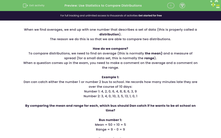# Use Statistics to Compare Distributions

In this worksheet, students will practise using an average and a measure of spread to describe a distribution.Key stage:  KS 4

Year:  GCSE

GCSE Subjects:   Maths

GCSE Boards:   AQA, Eduqas, Pearson Edexcel, OCR,

Curriculum topic:   Statistics

Curriculum subtopic:   Statistics Analysing Data

Difficulty level:#### Worksheet Overview

When we find averages, we end up with one number that describes a set of data (this is properly called a distribution).

The reason we do this is so that we are able to compare two distributions.

How do we compare?

To compare distributions, we need to find an average (this is normally the mean) and a measure of spread (for a small data set, this is normally the range).

When a question comes up in the exam, you need to make a comment on the average and a comment on the range.

Example 1:

Dan can catch either the number 1 or number 2 bus to school. He records how many minutes late they are over the course of 10 days:

Number 1: 4, 2, 0, 6, 4, 8, 8, 6, 3, 9

Number 2: 3, 4, 0, 10, 3, 5, 13, 1, 0, 1

By comparing the mean and range for each, which bus should Dan catch if he wants to be at school on time?

Bus number 1:

Mean = 50 ÷ 10 = 5

Range = 9 - 0 = 9

Bus number 2:

Mean = 40 ÷ 10 = 4

Range = 13 - 0 = 13

You could argue in favour of either bus:

The mean is lower for bus number 2, meaning that it is late less often.

The range is lower for bus number 1, meaning that bus 1 is more consistent and reliable.

Let's try some questions now.

### What is EdPlace?

We're your National Curriculum aligned online education content provider helping each child succeed in English, maths and science from year 1 to GCSE. With an EdPlace account you’ll be able to track and measure progress, helping each child achieve their best. We build confidence and attainment by personalising each child’s learning at a level that suits them.

Get started